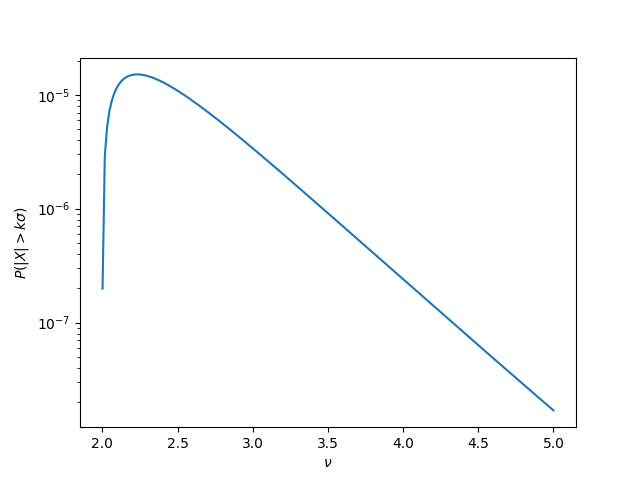# 50 sigma events for t distributions

I had a recent discussion with someone concerning a 50 sigma event, and that conversation prompted this post.

When people count “sigmas” they usually have normal distributions in mind. And six-sigma events so rare for normal random variables that it’s more likely the phenomena under consideration doesn’t have a normal distribution. As I explain here,

If you see a six-sigma event, that’s evidence that it wasn’t really a six-sigma event.

If six-sigma events are hard to believe, 50-sigma events are absurd. But not if you change the distribution assumption.

Chebyshev’s inequality says that if a random variable X has mean μ and variance σ², then

Prob( |X – μ| ≥ kσ) ≤ 1/k².

Not only that, it is possible to construct a random variable so that the inequality above is tight, i.e. it is possible to construct a random variable X such that the probability of it being 50 standard deviations away from its mean equals 1/2500. However, this requires constructing a custom random variable just to make equality hold. No distribution that you run into in the wild will have such a high probability of being that far out from its mean.

I wondered what the probability of a 50-sigma event would be like with a random variable with such a fat tail that it barely has a finite variance. I chose to look at Student t distributions because the variance of a Student t distribution with ν degrees of freedom is ν/(ν – 2), so the variance blows up as ν decreases toward 2. The smaller v us, the fatter the tails.

The probability of a t random variable with 3 degrees of freedom being 50 sigmas from its mean is about 3.4 × 10-6. The corresponding probability for 30 degrees of freedom is 6.7 × 10-31. So the former is rare but plausible, but the latter is so small as to be hard to believe.

This suggests that 50-sigma events are more likely with low degrees of freedom. And that’s sorta true, but not entirely. For 2.001 degrees of freedom, the probability of a 50-sigma event is 2 × 10-7, i.e. the probability is smaller for ν = 2.001 than for ν = 3.

Here’s a plot of the probability of 50-sigma events for a t distribution with ν degrees of freedom.Apparently the probability peaks somewhere around ν = 2.25 then decreases exponentially as ν increases.

This isn’t what I expected, but it makes sense after the fact. As ν increases, the tails get thinner, but σ also gets smaller. We have two competing effects. For ν less than 2.25 the size of σ matters more. For larger values of ν, the thinness of tails matters more.

## 2 thoughts on “50 sigma events for t distributions”

1. Diethard Michaelis

Brocken / missing here link in
“As I explain here”

2. Thanks. Just fixed it.